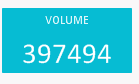# New to Qlik Sense

Discussion board where members can get started with Qlik Sense.

Announcements
QlikWorld, June 24-25, 2020. Free virtual event for DI and DA gurus. Register Now
cancel
Showing results for
Did you mean:
HighlightedCreator

## Number Formatting

I want to format the number in 'K' and 'M' depending on the values.

I am taking values into a text box.

Please find the screen shot attached.1 Solution

Accepted Solutions
HighlightedSpecialist II

## Re: Number Formatting

Try something like this

if(sum(value)/1000000000>1 , num(sum(value)/1000000000,'#,##0 B'),

if(sum(value)/1000000>1 , num(sum(value)/1000000,'#,##0 M'),

if(sum(value)/1000>1 , num(sum(value)/1000,'#,##0 K'),

num(sum(value),'#,##0'))))

4 Replies
HighlightedSpecialist

## Re: Number Formatting

I would suggest you use a KPI object instead you can set the number formatting to get and you will get the k or m

HighlightedSpecialist II

## Re: Number Formatting

Try something like this

if(sum(value)/1000000000>1 , num(sum(value)/1000000000,'#,##0 B'),

if(sum(value)/1000000>1 , num(sum(value)/1000000,'#,##0 M'),

if(sum(value)/1000>1 , num(sum(value)/1000,'#,##0 K'),

num(sum(value),'#,##0'))))

HighlightedSpecialist

## Re: Number Formatting

Sorry , Can't help you for K or B in text box, the above solutions works fine, if you are ready to compromise.

HighlightedCreator

## Re: Number Formatting

This Works.

I took my expression into a variable and then applied this if statement.

Thanks !